Next: Rotational coordinate transformations Up: Useful mathematics Previous: Vector identities

# Conservative fields

Consider a vector field. In general, the line integral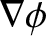depends on the path taken between the end points,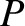and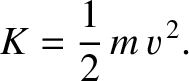. However, for some special vector fields the integral is path independent. Such fields are called conservative fields. It can be shown that if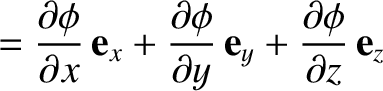is a conservative field then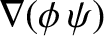for some scalar field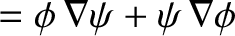. The proof of this is straightforward. Keepingfixed, we have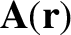(A.82)

where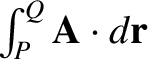is a well-defined function, due to the path-independent nature of the line integral. Consider moving the position of the end point by an infinitesimal amount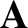in the-direction. We have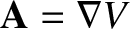(A.83)

Hence,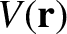(A.84)

with analogous relations for the other components of. It follows that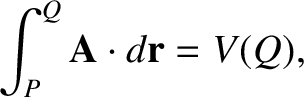(A.85)Next: Rotational coordinate transformations Up: Useful mathematics Previous: Vector identities
Richard Fitzpatrick 2016-03-31